Grade 6 Basic Geometrical Ideas Worksheets

Class 6 Maths Basic Geometrical Ideas Multiple Choice Questions (MCQs)

1. How many lines pass through one given point?
(a) one
(b) two
(c) countless
(d) none

2. How many lines pass through two given points?
(a) one
(b) two
(c) many
(d) none

3. The line segments forming a polygon are called ………………. .
(a) vertex
(b) sides
(c) angle
(d) curve

4. Two distinct lines meeting at a point are called ……………… .
(a) collinear lines
(b) intersecting lines
(c) parallel lines
(d) none of these

5. An angle is made up of two ……………….. starting from common end point.
(a) vertex
(b) lines
(c) rays
(d) line segments

6. A flat surface which extends indefinitely in all directions is called ……………… .
(a) line
(b) line segments
(c) plane
(d) point

7. Which of the following is pair of opposite sides in the given figure?
(a) AB, BC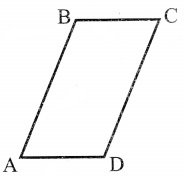8. Which of the following is the pair of adjacent angles in the given figure?
(a) ∠A, ∠ C
(b) ∠ B, ∠ D
(c) ∠ A, ∠ B
(d) none of these

9. A ………….. of a circle is a line segment joining any two points on the circle.
(b) diameter
(c) circumference
(d) chord

10. Three or more points lying on the same line are known as …………….. points.
(a) non-coi linear
(b) collinear
(c) intersecting
(d) none of these

11. A portion of a line which has two end points:
(a) line segment
(b) plane
(c) ray
(d) point

12. The number of sides in a pentagon are:
(a) 3
(b) 5
(c) 6
(d) 4

13. The lines which do not intersect and have equal distance between them are called:
(a) parallel lines
(b) perpendicular lines
(c) intersecting lines
(d) straight lines

14. Number of points a line can have are:
(a) infinite
(b) one
(c) two
(d) zero

15. A line segment AB is denoted as:
(a)$\overline{\mathrm{AB}}$
(b)$\underset { AB }{ \longleftrightarrow }$
(c)$\overrightarrow{\mathrm{AB}}$
(d) both a and c

16. If the length of a line segment AB = 3 cm then 2AB will be
(a) 8 cm
(b) 6 cm
(c) 4 cm
(d) 9 cm

17. Two line segments having the same length are said to be:
(a) same
(b) unequal
(c) parallel
(d) equal

18. The number of diagonal in a triangle are:
(a) 3
(b) 2
(c) 0
(d) 1

19. If two lines are perpendicular to each other then angle between them at the point of contact is:
(a) 80°
(b) 90°
(c) 85°
(d) 100°

20. A line segment has definite:
(b) length
(c) thickness
(d) area

Class 6 Maths Basic Geometrical Ideas True (T) or False (F)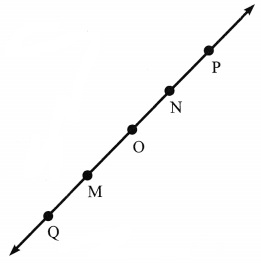1. Q, M, O, N, P are points on the line$\underset { MN }{ \longleftrightarrow }$.
2. M, O, N are points on a line segment$\overline{\mathrm{MN}}$.
3. M and N are end points of line segment$\overline{\mathrm{MN}}$.
4. O and N are end points of line segment$\overline{\mathrm{OP}}$.
5. M is one of the end points of line segment$\overline{\mathrm{QO}}$.
6. M is point on ray$\overrightarrow{\mathrm{OP}}$.
7. Ray$\overrightarrow{\mathrm{OP}}$ is different from ray$\overrightarrow{\mathrm{QP}}$.
8. Ray$\overrightarrow{\mathrm{OP}}$ is same as ray$\overrightarrow{\mathrm{OM}}$.
9. Ray$\overrightarrow{\mathrm{OM}}$ is not opposite to ray$\overrightarrow{\mathrm{OP}}$.
10. N is the initial point of$\overrightarrow{\mathrm{NP}}$ and$\overrightarrow{\mathrm{NM}}$.

Class 6 Maths Basic Geometrical Ideas Very Short Answer Type Questions

1. Join the points given below.How many line segments do you get?
2. Which of the following has a fixed length?
(a) a line
(b) a line segment
(c) a ray
3. Draw a polygon having 3 sides
4. Name the rays in the following figure. What is the starting point of each ray?5. Count and name all the angles in the adjacent figure.6. Draw one open curve and one closed curve.

Class 6 Maths Basic Geometrical Ideas Short Answer Type Questions

1.
(a) Identify the number of triangles in the given figure.
(b) Write the names of all triangles.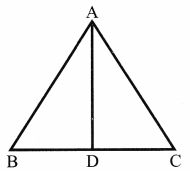2. Draw a circle and show
(a) its centre
(b) a sector
(d) a segment

3. Draw figures to show
(a) Point M lies on the line PQ
(b) AB and CD intersect at P

4. Draw any triangles and locate
(a) Point A in its interior
(b) Point B in its exterior
(c) Point C on it

Class 6 Maths Basic Geometrical Ideas Long Answer Type Questions

1. Use the figure to name:
(a) Line containing point E
(b) Line passing through A
(c) Line on which O lies
(d) Two pairs of intersecting lines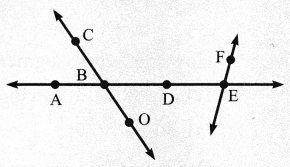2.
(a) Identify three triangles in the figure
(b) Write the names of six line segments
(c) Which two triangles have ∠Q as common?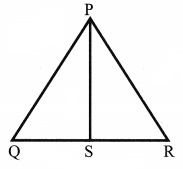3. Draw a quadrilateral KLMN and write:
(a) two pairs of adjacent sides
(b) two pairs of adjacent angles
(c) two pairs of opposite angles